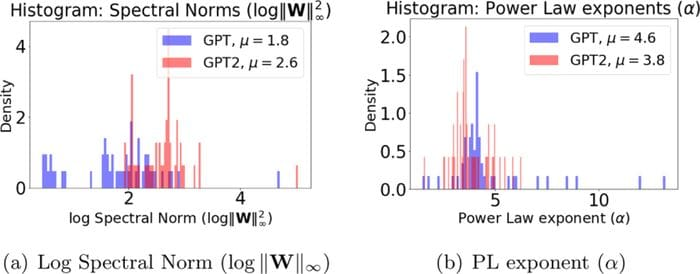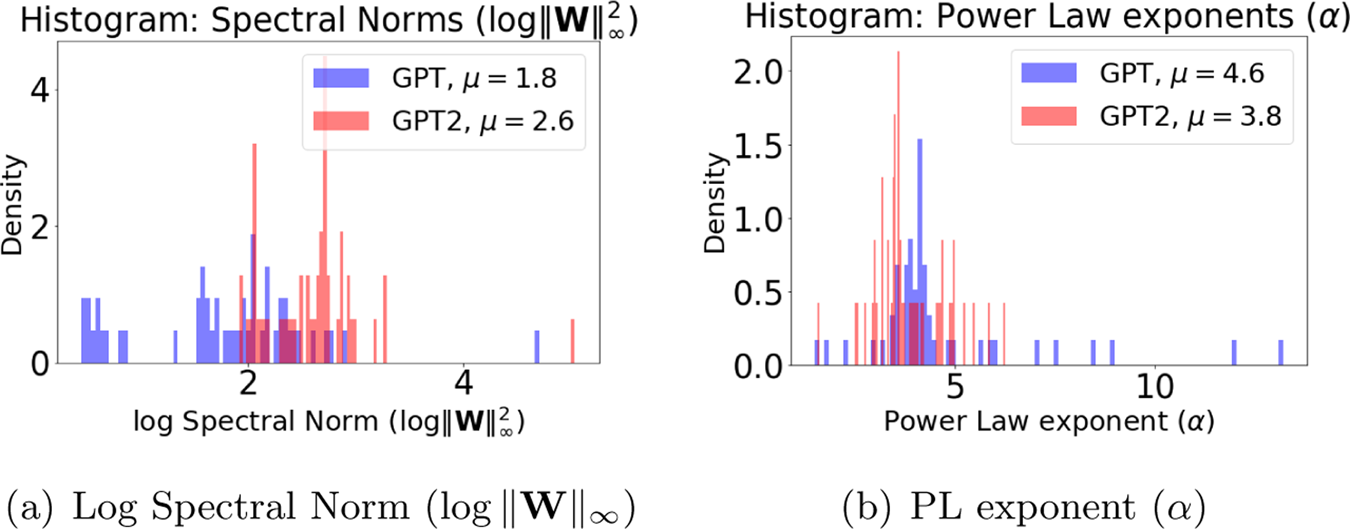# How to Tell if You Have Trained Your Model with Enough Data

WeightWatcher is an open-source, diagnostic tool for evaluating the performance of (pre)-trained and fine-tuned Deep Neural Networks. It is based on state-of-the-art research into Why Deep Learning Works.

By Charles Martin, Calculation ConsultingDeep Neural Networks (DNN) require a lot of training data. Even fine-tuning a model can require a lot. A LOT. So how can you know if you have used enough? For Computer Vision (CV) models, you can always look at the test error. But what about fine-tuning large, transformer models like BERT or GPT?

• What is the best metric to evaluate your model ?
• How can you be sure you trained it with enough data ?
• And how can your customers be sure ?

WeightWatcher can help.

pip install weightwatcher

WeightWatcher is an open-source, diagnostic tool for evaluating the performance of (pre)-trained and fine-tuned Deep Neural Networks. It is based on state-of-the-art research into Why Deep Learning Works. Recently, it has been featured in Nature:Here, we show you how to use WeightWatcher to determine if your DNN model has been trained with enough data.

In the paper, we consider the example of GPT vs GPT2. GPT is a NLP Transformer model, developed by OpenAI, to generate fake text. When it was first developed, OpenAI released the GPT model, which had specifically been trained with a small data set, making it unusable to generate fake text. Later, they realized fake text is good business, and they released GPT2, which is just like GPT. but trained with enough data to make it useful.We can apply WeightWatcher to GPT and GPT2 and compare the results; we will see that the WeightWatcher log spectral norm and alpha (power law) metrics can immediately tell us that something is wrong with the GPT model. This is shown in Figure 6 of the paper;Here we will walk through exactly how to do this yourself for the WeightWatcher Power Law (PL) alpha metric$(\alpha)$, and explain how to interpret these plots.

It is recommended to run these calculations in a Jupiter notebook, or Google Colab. (For reference, you can also view the actual notebook used to create the plots in the paper, however, this uses an older version of weightwatcher)

For this post, we provide a working notebook in the WeightWatcher github repo.

WeightWatcher understands the basic Huggingface models. Indeed, WeightWatcher supports:

• TF2.0 / Keras
• pyTorch 1.x
• HuggingFace

and (soon)

ONNX (in the current trunk)

Currently, we support Dense and Conv2D layers. Support for more layers is coming. For our NLP Transformer models, we only need support for the Dense layers.

First, we need the GPT and GPT2 pyTorch models. We will use the popular HuggingFace transformers package.

!pip install transformers

Second, we need to import pyTorch and weightwatcher

import torch
import weightwatcher as ww

We will also want the pandas and matplotlib libraries to help us interpret the weightwatcher metrics. In Jupyter notebooks, this looks like

import pandas as pd

import matplotlib
import matplotlib.pyplot as plt
%matplotlib inline

We now import the transformers package and the 2 model classes

import transformers
from transformers import OpenAIGPTModel,GPT2Model

We have to get the 2 pretrained models, and run model.eval()

gpt_model = OpenAIGPTModel.from_pretrained('openai-gpt')
gpt_model.eval();

gpt2_model = GPT2Model.from_pretrained('gpt2')
gpt2_model.eval();

To analyze our GPT models with WeightWatcher , simply create a watcher instance, and run watcher.analyze(). This will return a pandas dataframe with the metrics for each layer

watcher = ww.WeightWatcher(model=gpt_model)
gpt_details = watcher.analyze()

The details dataframes reports quality metrics that can be used to analyze the model performance–without needing access to test or training data. The most important metric is our Power Law metric$\alpha$. WeightWatcher reports$\alpha$ for every layer. The GPT model has nearly 50 layers, so it is convenient to examine all the layer alphas at once as a histogram (using the pandas API).

gpt_details.alpha.plot.hist(bins=100, color='red', alpha=0.5, density=True, label='gpt')
plt.xlabel(r"alpha $(\alpha)$ PL exponent")
plt.legend()

This plots the density of the$\alpha$ values for all layers in the GPT model.From this histogram, we can immediately see 2 problems with the model

• The peak$\alpha\sim 4$. which is higher than optimal for a well trained model.
• There are several outliers with$\alpha>6$, indicating several poorly trained layers.

So knowing nothing about GPT, and having never seen the test or training data, WeightWatcher tells us that this model should never go into production.

Now let’s look GPT2, which has the same architecture, but trained with more and better data. Again, we make a watcher instance with the model specified, and just run watcher.analyze()

watcher = ww.WeightWatcher(model=gpt2_model)
gpt2_details = watcher.analyze()

Now let’s compare the Power Law alpha metrics for GPT and GPT2. We just create 2 histograms, 1 for each model, and overlay them.

gpt_details.alpha.plot.hist(bins=100, color='red', alpha=0.5, density=True, label='gpt')
gpt2_details.alpha.plot.hist(bins=100, color='green', density=True, label='gpt2')
plt.xlabel(r"alpha $(\alpha)$ PL exponent")
plt.legend()

The layer alphas for GPT are shown in red, and for GPT2 in green, and the histograms differ significantly. For the GPT2, the peak $alpha\sim 3.5&bg=ffffff$ and, more importantly, there are no outlier $latex \alpha>6&bg=ffffff$ Smaller alphas are better, and the GPT2 model is much better than GPT because it is trained with significantly more and better data.• Help you decide if you have trained it with enough data (as shown here)
• Detect potential layers that are overtrained (as shown in a previous blog)
• Be used to get early stopping criteria (when you can’t peek at the test data)
• Predict trends in the test accuracies across models and hyperparameters (see our Nature paper, and our most recent submission).

and many other things.

Please give it a try. And if it is useful to you, let me know.

And if your company needs help with AI, reach out. I provide strategy consulting, mentorship, and hands-on development. #talkToChuck, #theAIguy.

Bio: Dr. Charles Martin has developed the weight watcher project as a joint research effort with UC Berkeley. He runs a boutique consultancy in San Francisco, CA, helping clients develop AI solutions. He has worked with large companies like eBay, Blackrock, GoDaddy, and Walmart as well as very successful startups such as Aardvark (acquired by Google) and Demand Media (the first Billion dollar IPO since Google). He also advises the Page family offices, through the Anthropocene Institute, on areas of AI, nuclear physics, and quantum mechanics/computing.

Original. Reposted with permission.

Related: